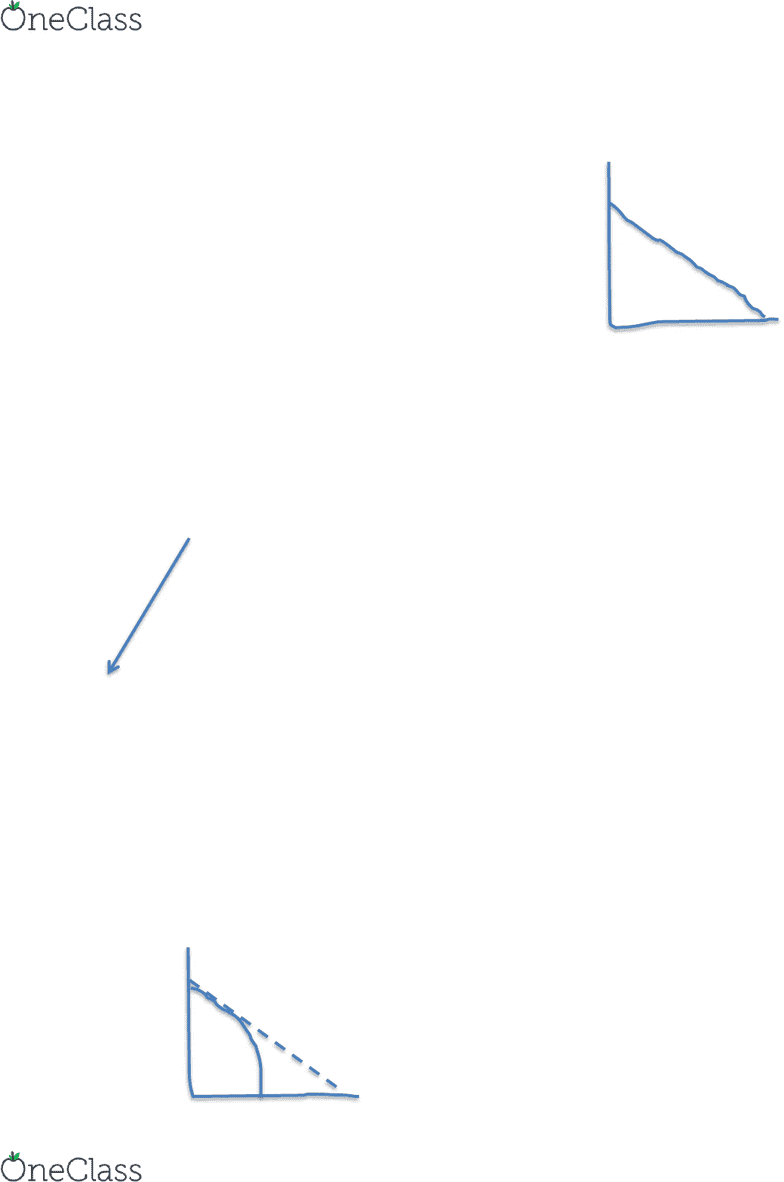# ECON 2610 Lecture Notes - Lecture 3: Demand Curve, Perfect Competition, Indifference Curve

33 views3 pages
School
Department
Course

For unlimited access to Class Notes, a Class+ subscription is required.Week 3 Notes
The Standard Trade Model
C
Smith/Ricardo
Qc = f(Labor)
Assume PPF
o Constant returns to scale
o Markets are perfectly competitive
EX: on PPF, 1C = 2F, it will not change
F
Modern Trade Model
Qc = f((k)apital and (L)abor)
Production of C and F can take 2 options
o C and F use k and L in the exact same proportion
C = (k/L) = 4/1
F = (k/L) = 4/1
We get the same outcome
PPF is straight line
o C and F use k and L in different proportions
C = (k/L) = 4/1
F = (k/L) = 1/1
PPF assumes all inputs are fully employed
If we keep this assumption, but C and F are different,
then we have a problem
By producing less C, it will free up capital, but then it just sits there and the owners
of capital no longer make money
If price falls low enough, they will use it for other reasons
We underutilize capital
o Resources are freed up and then not needed
When this happens, the PPF curves
o We begin to produce more F, so Pk falls as curve falls
o Give it enough time, and an alternative use for C will be found
No room for classes at DU, we will use a restaurant if cheap
enough
o At first, we will have too much capital with no use
C
A
B
find more resources at oneclass.com
find more resources at oneclass.com
Unlock document

This preview shows page 1 of the document.
Unlock all 3 pages and 3 million more documents.

Already have an account? Log in

# Get access

Grade+
\$10 USD/m
Billed \$120 USD annually
Homework Help
Class Notes
Textbook Notes
40 Verified Answers
Study Guides
1 Booster Class
Class+
\$8 USD/m
Billed \$96 USD annually
Homework Help
Class Notes
Textbook Notes
30 Verified Answers
Study Guides
1 Booster Class